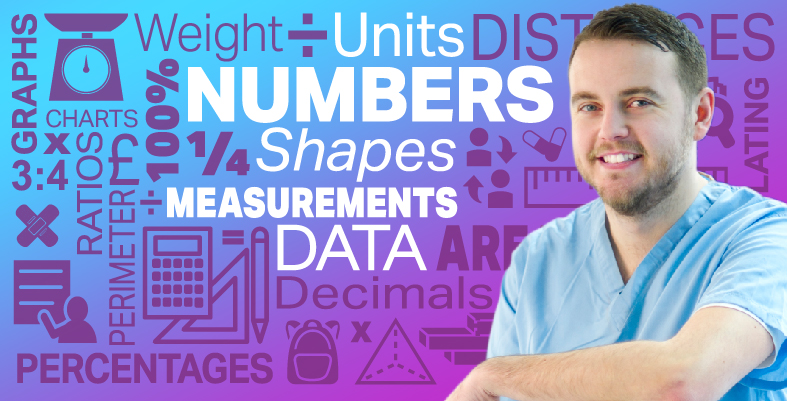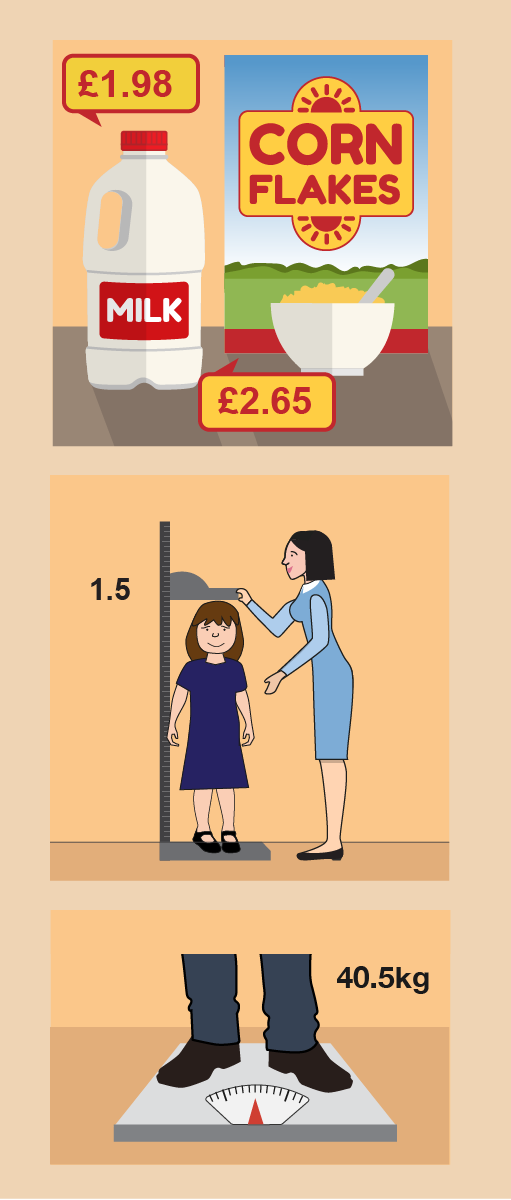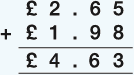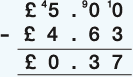Everyday maths for Health and Social Care and Education Support 1

Start this free course now. Just create an account and sign in. Enrol and complete the course for a free statement of participation or digital badge if available.

Free course

# 4.2 Calculations using decimals

When you make any calculation with decimals – that is, addition, subtraction, multiplication and division – you may use a calculator. When using a calculator it is very important to make sure that the decimal point is in the correct place. If you don’t, you’ll get the wrong answer.

Now try the following activity. Remember to check your answers once you have completed the questions.

## Activity 13: Using decimalsFigure 19 Using decimals
1. You buy a box of corn flakes for £2.65 and a bottle of milk for £1.98.
• a.What is the total cost of these items?
• b.You pay for them with a £5 note. How much change should you get?
2. A patient’s height and weight are used to calculate their Body Mass Index (BMI). These measures are often decimal numbers.
• a.What is the patient’s weight in the image above?
• b.What is the patient’s height (in metres) in the image above? Multiply the height by itself.
3. Convert 6.25 m to cm.

1. The answers are as follows:
• a.Add the cost of the two items:(Keep the decimal points in line.)

The total cost of the items is £4.63.

• b.Take away the total cost from £5:You should get 37p change from £5.

2.

• a.The patient's weight is 40.5 kg
• b.The patient’s height is 1.5 m. Therefore 1.5 × 1.5 = 2.25
• c.40.5 ÷ 2.25 = 18.

So the BMI of the patient is 18.

3. To convert 6.25 m into cm, you need to multiply the amount by 100.

• 6.25 × 100 = 625

So the answer is 625 cm.

## Summary

In this section you have learned about how:

• the value of a digit depends on its position in a decimal number
• to approximate answers to calculations involving decimal numbers
• to add, subtract, multiply and divide using decimal numbers.

This will help when working with money and measurements.

FSM_SSH_1